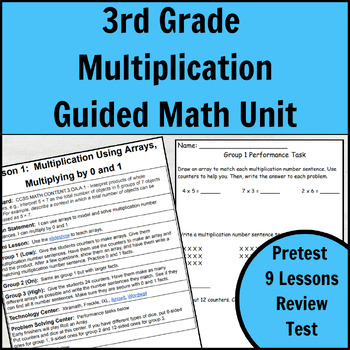# Multiplication Guided Math LessonsResource Type
File Type

PDF

(889 KB|121 pages)
Standards
Also included in:
1. Guided math is the best way to differentiate your math lessons. Use these Money Guided Math Lessons to meet the needs of ALL your students.This product includes detailed lesson plans with:-A minilesson with an introductory video-Differentiated plans for a Meet with the Teacher Center-3 differentiate
\$48.00
\$43.20
Save \$4.80
• Product Description
• StandardsNEW

Guided math is the best way to differentiate your math lessons. Use these Multiplication Guided Math Lessons to meet the needs of ALL your students.

This product includes 9 detailed lesson plans:

-Lesson 1: Multiplication Using Arrays, Multiplying by 0 and 1

-Lesson 2: Multiplication with Skip Counting, Multiplying by 5 and 10

-Lesson 3: Multiplication with Repeated Addition, Multiplying by 2 and 4

-Lesson 4: The Commutative Property of Multiplication, Multiplying by 3 and 6

-Lesson 5: The Distributive Property of Multiplication, Multiplying by 7 and 8

-Lesson 6: Using the Multiplication Table, Multiplying by 9

-Lesson 7: Multiplying by Multiples of 10

-Lesson 8: The Associative Property of Multiplication

-Lesson 9: Multiplication Problem Solving

Each lesson includes:

-A minilesson with an introductory video

-Differentiated plans for a Meet with the Teacher Center

-3 differentiated levels for a Problem Solving Center with extra time activities

-Websites to use for a Technology Center

- An exit ticket to assess student mastery

This product also includes:

-A review to check for understanding

-The Basic Multiplication Unlock the Box Mystery for extra practice

-A summative end of unit test

Check out my other guided math products:

Place Value and Rounding Guided Math Unit

Money Guided Math Unit

Addition and Subtraction Guided Math Unit

Get all the guided math units in this bundle.

This post tells more about how I run my guided math groups.

Identify arithmetic patterns (including patterns in the addition table or multiplication table), and explain them using properties of operations. For example, observe that 4 times a number is always even, and explain why 4 times a number can be decomposed into two equal addends.
Fluently multiply and divide within 100, using strategies such as the relationship between multiplication and division (e.g., knowing that 8 × 5 = 40, one knows 40 ÷ 5 = 8) or properties of operations. By the end of Grade 3, know from memory all products of two one-digit numbers.
Apply properties of operations as strategies to multiply and divide. Examples: If 6 × 4 = 24 is known, then 4 × 6 = 24 is also known. (Commutative property of multiplication.) 3 × 5 × 2 can be found by 3 × 5 = 15, then 15 × 2 = 30, or by 5 × 2 = 10, then 3 × 10 = 30. (Associative property of multiplication.) Knowing that 8 × 5 = 40 and 8 × 2 = 16, one can find 8 × 7 as 8 × (5 + 2) = (8 × 5) + (8 × 2) = 40 + 16 = 56. (Distributive property.)
Use multiplication and division within 100 to solve word problems in situations involving equal groups, arrays, and measurement quantities, e.g., by using drawings and equations with a symbol for the unknown number to represent the problem.
Interpret products of whole numbers, e.g., interpret 5 × 7 as the total number of objects in 5 groups of 7 objects each. For example, describe a context in which a total number of objects can be expressed as 5 × 7.
Total Pages
121 pages
Included
Teaching Duration
3 Weeks
Report this Resource to TpT
Reported resources will be reviewed by our team. Report this resource to let us know if this resource violates TpT’s content guidelines.